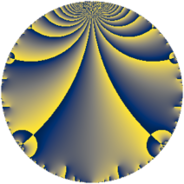# Properties

 Label 1859.4.aLevel $1859$ Weight $4$ Character orbit 1859.a Rep. character $\chi_{1859}(1,\cdot)$ Character field $\Q$ Dimension $388$ Newform subspaces $17$ Sturm bound $728$ Trace bound $5$

# Related objects

## Defining parameters

 Level: $$N$$ $$=$$ $$1859 = 11 \cdot 13^{2}$$ Weight: $$k$$ $$=$$ $$4$$ Character orbit: $$[\chi]$$ $$=$$ 1859.a (trivial) Character field: $$\Q$$ Newform subspaces: $$17$$ Sturm bound: $$728$$ Trace bound: $$5$$ Distinguishing $$T_p$$: $$2$$

## Dimensions

The following table gives the dimensions of various subspaces of $$M_{4}(\Gamma_0(1859))$$.

Total New Old
Modular forms 560 388 172
Cusp forms 532 388 144
Eisenstein series 28 0 28

The following table gives the dimensions of the cuspidal new subspaces with specified eigenvalues for the Atkin-Lehner operators and the Fricke involution.

$$11$$$$13$$FrickeDim.
$$+$$$$+$$$$+$$$$102$$
$$+$$$$-$$$$-$$$$93$$
$$-$$$$+$$$$-$$$$88$$
$$-$$$$-$$$$+$$$$105$$
Plus space$$+$$$$207$$
Minus space$$-$$$$181$$

## Trace form

 $$388q - 2q^{2} - 2q^{3} + 1544q^{4} - 14q^{5} + 34q^{6} - 20q^{7} - 72q^{8} + 3554q^{9} + O(q^{10})$$ $$388q - 2q^{2} - 2q^{3} + 1544q^{4} - 14q^{5} + 34q^{6} - 20q^{7} - 72q^{8} + 3554q^{9} + 58q^{10} - 22q^{11} - 244q^{12} + 212q^{14} - 18q^{15} + 6320q^{16} - 36q^{17} - 428q^{18} - 160q^{19} - 160q^{20} - 92q^{21} + 22q^{22} + 222q^{23} + 732q^{24} + 9470q^{25} + 634q^{27} - 100q^{28} - 120q^{29} - 474q^{30} - 22q^{31} - 508q^{32} + 22q^{33} - 1108q^{34} - 308q^{35} + 14000q^{36} - 122q^{37} + 1144q^{38} + 1164q^{40} - 8q^{41} + 1952q^{42} - 188q^{43} - 528q^{44} + 908q^{45} + 306q^{46} + 540q^{47} - 76q^{48} + 18924q^{49} + 2660q^{50} - 548q^{51} - 592q^{53} + 3978q^{54} + 418q^{55} + 708q^{56} + 2344q^{57} + 940q^{58} - 606q^{59} + 3700q^{60} - 2512q^{61} - 1234q^{62} - 2688q^{63} + 29068q^{64} - 286q^{66} + 450q^{67} - 592q^{68} + 2586q^{69} + 88q^{70} - 890q^{71} - 1844q^{72} + 40q^{73} + 3214q^{74} - 2992q^{75} + 304q^{76} + 396q^{77} + 724q^{79} + 1832q^{80} + 29988q^{81} - 3040q^{82} + 1788q^{83} + 10436q^{84} - 340q^{85} + 6188q^{86} - 4424q^{87} - 132q^{88} - 2990q^{89} + 732q^{90} + 1164q^{92} - 886q^{93} + 72q^{94} - 696q^{95} - 2068q^{96} - 2194q^{97} - 1990q^{98} - 484q^{99} + O(q^{100})$$

## Decomposition of $$S_{4}^{\mathrm{new}}(\Gamma_0(1859))$$ into newform subspaces

Label Dim. $$A$$ Field CM Traces A-L signs $q$-expansion
$$a_2$$ $$a_3$$ $$a_5$$ $$a_7$$ 11 13
1859.4.a.a $$2$$ $$109.685$$ $$\Q(\sqrt{3})$$ None $$-2$$ $$-2$$ $$-2$$ $$-20$$ $$-$$ $$+$$ $$q+(-1+\beta )q^{2}+(-1+4\beta )q^{3}+(-4+\cdots)q^{4}+\cdots$$
1859.4.a.b $$4$$ $$109.685$$ 4.4.297133.1 None $$0$$ $$-4$$ $$6$$ $$17$$ $$-$$ $$+$$ $$q-\beta _{1}q^{2}+(-1-\beta _{3})q^{3}+(2+\beta _{1}+2\beta _{2}+\cdots)q^{4}+\cdots$$
1859.4.a.c $$6$$ $$109.685$$ $$\mathbb{Q}[x]/(x^{6} - \cdots)$$ None $$6$$ $$-6$$ $$8$$ $$53$$ $$+$$ $$+$$ $$q+(1-\beta _{1})q^{2}+(-1-\beta _{4})q^{3}+(4-\beta _{1}+\cdots)q^{4}+\cdots$$
1859.4.a.d $$9$$ $$109.685$$ $$\mathbb{Q}[x]/(x^{9} - \cdots)$$ None $$0$$ $$8$$ $$-30$$ $$-25$$ $$-$$ $$+$$ $$q-\beta _{1}q^{2}+(1-\beta _{3})q^{3}+(5-\beta _{2}-\beta _{3}+\cdots)q^{4}+\cdots$$
1859.4.a.e $$11$$ $$109.685$$ $$\mathbb{Q}[x]/(x^{11} - \cdots)$$ None $$-6$$ $$6$$ $$4$$ $$-45$$ $$+$$ $$+$$ $$q+(-1+\beta _{1})q^{2}+(1-\beta _{5})q^{3}+(6-\beta _{1}+\cdots)q^{4}+\cdots$$
1859.4.a.f $$17$$ $$109.685$$ $$\mathbb{Q}[x]/(x^{17} - \cdots)$$ None $$-4$$ $$-6$$ $$-16$$ $$6$$ $$-$$ $$+$$ $$q-\beta _{1}q^{2}-\beta _{8}q^{3}+(5+\beta _{2})q^{4}+(-1+\cdots)q^{5}+\cdots$$
1859.4.a.g $$17$$ $$109.685$$ $$\mathbb{Q}[x]/(x^{17} - \cdots)$$ None $$0$$ $$-6$$ $$-24$$ $$-62$$ $$-$$ $$+$$ $$q-\beta _{1}q^{2}-\beta _{6}q^{3}+(3+\beta _{2})q^{4}+(-1+\cdots)q^{5}+\cdots$$
1859.4.a.h $$17$$ $$109.685$$ $$\mathbb{Q}[x]/(x^{17} - \cdots)$$ None $$0$$ $$-6$$ $$24$$ $$62$$ $$+$$ $$+$$ $$q+\beta _{1}q^{2}-\beta _{6}q^{3}+(3+\beta _{2})q^{4}+(1-\beta _{7}+\cdots)q^{5}+\cdots$$
1859.4.a.i $$17$$ $$109.685$$ $$\mathbb{Q}[x]/(x^{17} - \cdots)$$ None $$4$$ $$-6$$ $$16$$ $$-6$$ $$+$$ $$+$$ $$q+\beta _{1}q^{2}-\beta _{8}q^{3}+(5+\beta _{2})q^{4}+(1+\beta _{6}+\cdots)q^{5}+\cdots$$
1859.4.a.j $$18$$ $$109.685$$ $$\mathbb{Q}[x]/(x^{18} - \cdots)$$ None $$-2$$ $$0$$ $$-20$$ $$-28$$ $$+$$ $$-$$ $$q-\beta _{1}q^{2}-\beta _{5}q^{3}+(4+\beta _{2})q^{4}+(-1+\cdots)q^{5}+\cdots$$
1859.4.a.k $$18$$ $$109.685$$ $$\mathbb{Q}[x]/(x^{18} - \cdots)$$ None $$2$$ $$0$$ $$20$$ $$28$$ $$-$$ $$-$$ $$q+\beta _{1}q^{2}-\beta _{5}q^{3}+(4+\beta _{2})q^{4}+(1+\beta _{11}+\cdots)q^{5}+\cdots$$
1859.4.a.l $$36$$ $$109.685$$ None $$-4$$ $$12$$ $$-40$$ $$-56$$ $$+$$ $$-$$
1859.4.a.m $$36$$ $$109.685$$ None $$4$$ $$12$$ $$40$$ $$56$$ $$-$$ $$-$$
1859.4.a.n $$39$$ $$109.685$$ None $$0$$ $$-23$$ $$-23$$ $$4$$ $$-$$ $$+$$
1859.4.a.o $$39$$ $$109.685$$ None $$0$$ $$-23$$ $$23$$ $$-4$$ $$+$$ $$-$$
1859.4.a.p $$51$$ $$109.685$$ None $$0$$ $$21$$ $$-41$$ $$-4$$ $$+$$ $$+$$
1859.4.a.q $$51$$ $$109.685$$ None $$0$$ $$21$$ $$41$$ $$4$$ $$-$$ $$-$$

## Decomposition of $$S_{4}^{\mathrm{old}}(\Gamma_0(1859))$$ into lower level spaces

$$S_{4}^{\mathrm{old}}(\Gamma_0(1859)) \cong$$ $$S_{4}^{\mathrm{new}}(\Gamma_0(11))$$$$^{\oplus 3}$$$$\oplus$$$$S_{4}^{\mathrm{new}}(\Gamma_0(13))$$$$^{\oplus 4}$$$$\oplus$$$$S_{4}^{\mathrm{new}}(\Gamma_0(143))$$$$^{\oplus 2}$$$$\oplus$$$$S_{4}^{\mathrm{new}}(\Gamma_0(169))$$$$^{\oplus 2}$$